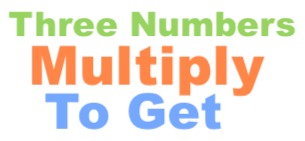Three Numbers Multiply to Get CalculatorWelcome to the Three Numbers Multiply to Get Calculator. The Three Numbers Multiply to Get Calculator can calculate all the combinations of 3 numbers that when multiplied equal the number of your choice.

Please enter your number below to see all combinations of 3 numbers that when multiplied together equal your number.

Here are some examples of what our calculator can answer:

What three numbers multiply to get 512?

What three numbers multiply to get 72?

What three numbers multiply to get 36?

What three numbers multiply to get 64?

What three numbers multiply to get 120?

What three numbers multiply to get 96?

What three numbers multiply to get 168?

What three numbers multiply to get 343?

What three numbers multiply to get 60?

What three numbers multiply to get 18?